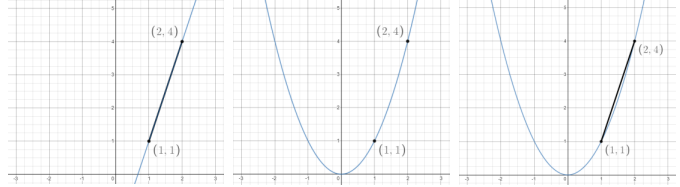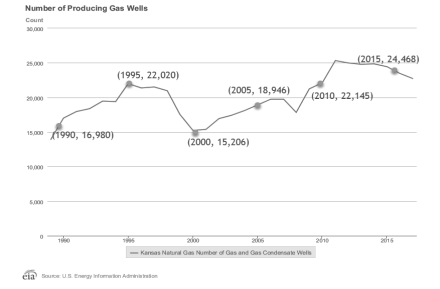# MA.912.F.1.3Export Print
Calculate and interpret the average rate of change of a real-world situation represented graphically, algebraically or in a table over a specified interval.

### Clarifications

Clarification 1: Instruction includes making the connection to determining the slope of a particular line segment.
General Information
Subject Area: Mathematics (B.E.S.T.)
Strand: Functions
Status: State Board Approved

## Benchmark Instructional Guide

### Terms from the K-12 Glossary

• Rate of Change
• Slope

### Vertical Alignment

Previous Benchmarks

Next Benchmarks

### Purpose and Instructional Strategies

In grade 8, students determined the slope of a linear equation in two variables and analyzed graphical representations of functional relationships. In Algebra I, students calculate the average rate of change in real-world situations represented in various ways. In later courses, this concept leads to the difference quotient and differential calculus.
• The purpose of this benchmark is to extend students’ understanding of rate of change to allow them to apply it in non-linear contexts.
• Instruction emphasizes a graphical context so students can see the meaning of the average rate of change. Students can use graphing technology to help visualize this.
• Starting with the linear function $f$($x$) = 3$x$ − 2, shown below, ask students to calculate the rate of change between two points using the slope formula. Lead students to verify their calculations visually.
• Once students have successfully used the formula, transition to the graph of $f$($x$) = $x$2
• Highlight the same two points and ask students to discuss what the rate of change might be between them (MTR.4.1). Lead students realize that while there is not a constant rate of change, they can calculate an average rate of change for an interval. Show students that this is equivalent to calculating the slope of the line segment that connects the two points of interest.• Once students have an understanding, ask them to find the average rate of change for other intervals, such as −2 ≤ $x$ ≤ −1 or 0 ≤ $x$ ≤ 2. As each of these calculations produce different values, reinforce the concept that non-linear functions do not have constant rates of change (MTR.5.1).
• Look for opportunities to continue students’ work with function notation. Ask students to find the average rate of change between $f$(1) and $f$(4) for $f$($x$).

### Common Misconceptions or Errors

• Some graphs presented to students will only display a certain interval of data. Some students may mistakenly interpret the rate of change for that entire interval rather than a given sub-interval. In these cases, lead students to highlight the domain and range for the sub-interval requested so they can see it more clearly.
• Students may be confused if the average rate of change is 0, even though the function is not constant.
• For example, the average rate of change of the function $f$($x$) =  $x$2 from $x$ = −1 to $x$ = 1 is 0.

### Strategies to Support Tiered Instruction

• When determining an average rate of change on an interval where the function only increases or only decreases, instruction includes directions to highlight the domain and range for the interval so that the change can be seen more clearly.
• Instruction includes providing the graph to visualize the change in the $x$- and $y$-values by drawing a line from the leftmost point on the graph within the interval to the rightmost point on the graph within the interval. Discuss with students how the average rate of change is the slope of the line between the two points.
• For example, for the function $f$($x$) = $x$, on the interval [−2, 2], the average rate of change is zero. This can be visualized by drawing a line from the point (−2, 4) to (2, 4) which has slope of zero (horizontal line).
• Instruction includes assistance recognizing the connection between slope (MA.912.AR.2) and rate of change for a linear function. A linear function has a constant rate of change. Regardless of the interval, the rate of change is the same (constant). For nonlinear functions rate of change is not constant, so it is not considered slope. However, the formula for slope can be used to calculate the average rate of change over an interval.
• Teacher provides instruction on assigning the ordered pairs when calculating average rate of change. The formula for slope is the change in $y$ divided by the change in $x$. The designation of the interval points as the first or second pair of coordinates does not matter.
• Teacher co-creates an $x$-$y$ chart to organize information when solving real-world problems involving a graphical representation. The $x$ column should be labeled with the input description used for the $x$-axis. The $y$ column should be labeled with the output description used for the $y$-axis.

• Jorge invests an amount of \$5,000 in a money market account at an annual interest rate of 5%, compounded monthly. The function $f$($x$) = 5000(1 + $\frac{\text{0.05}}{\text{12}}$)12$x$, represents the value of the investment after $x$ years.
• Part A. Find the average rate of change in the value of Jorge’s investment between year 5 and year 7, between year 10 and year 12, and between year 15 and year 17.
• Part B. What do you notice about the change in value of the investment over each interval?

### Instructional Items

Instructional Item 1
• The graph below represents the number of producing gas wells in Kansas from 1989 to 2017. What is the average rate of change between 2000 and 2015?*The strategies, tasks and items included in the B1G-M are examples and should not be considered comprehensive.

## Related Courses

This benchmark is part of these courses.
1200310: Algebra 1 (Specifically in versions: 2014 - 2015, 2015 - 2022, 2022 and beyond (current))
1200320: Algebra 1 Honors (Specifically in versions: 2014 - 2015, 2015 - 2022, 2022 and beyond (current))
1200370: Algebra 1-A (Specifically in versions: 2014 - 2015, 2015 - 2022, 2022 and beyond (current))
1200380: Algebra 1-B (Specifically in versions: 2014 - 2015, 2015 - 2022, 2022 and beyond (current))
1200400: Foundational Skills in Mathematics 9-12 (Specifically in versions: 2014 - 2015, 2015 - 2022, 2022 and beyond (current))
7912080: Access Algebra 1A (Specifically in versions: 2014 - 2015, 2015 - 2018, 2018 - 2019, 2019 - 2022, 2022 and beyond (current))
7912090: Access Algebra 1B (Specifically in versions: 2014 - 2015, 2015 - 2018, 2018 - 2019, 2019 - 2022, 2022 and beyond (current))
1200315: Algebra 1 for Credit Recovery (Specifically in versions: 2014 - 2015, 2015 - 2022, 2022 and beyond (current))
1200375: Algebra 1-A for Credit Recovery (Specifically in versions: 2014 - 2015, 2015 - 2022, 2022 and beyond (current))
1200385: Algebra 1-B for Credit Recovery (Specifically in versions: 2014 - 2015, 2015 - 2022, 2022 and beyond (current))
7912075: Access Algebra 1 (Specifically in versions: 2014 - 2015, 2015 - 2018, 2018 - 2019, 2019 - 2022, 2022 and beyond (current))
1700600: GEAR Up 1 (Specifically in versions: 2020 - 2022, 2022 and beyond (current))
1700610: GEAR Up 2 (Specifically in versions: 2020 - 2022, 2022 and beyond (current))
1700620: GEAR Up 3 (Specifically in versions: 2020 - 2022, 2022 and beyond (current))
1700630: GEAR Up 4 (Specifically in versions: 2020 - 2022, 2022 and beyond (current))
1200710: Mathematics for College Algebra (Specifically in versions: 2022 and beyond (current))
1209315: Mathematics for ACT and SAT (Specifically in versions: 2022 and beyond (current))

## Related Access Points

Alternate version of this benchmark for students with significant cognitive disabilities.
MA.912.F.1.AP.3: Given a real-world situation represented graphically or algebraically, identify the rate of change as positive, negative, zero or undefined.

## Related Resources

Vetted resources educators can use to teach the concepts and skills in this benchmark.

## Formative Assessments

Pizza Palace:

Students are given a table of functional values in context and are asked to find the average rate of change over a specific interval.

Type: Formative Assessment

Air Cannon:

Students are given a graph of an exponential function and are asked to calculate and compare the average rate of change over two different intervals of time.

Type: Formative Assessment

Estimating the Average Rate of Change:

Students are asked to estimate the average rate of change of a nonlinear function over two different intervals given its graph.

Type: Formative Assessment

Identifying Rate of Change:

Students are asked to calculate and interpret the rate of change of a linear function given its graph.

Type: Formative Assessment

## Lesson Plans

How Fast Do Objects Fall?:

Students will investigate falling objects with very low air friction.

Type: Lesson Plan

Which Function?:

This activity has students apply their knowledge to distinguish between numerical data that can be modeled in linear or exponential forms. Students will create mathematical models (graph, equation) that represent the data and compare these models in terms of the information they show and their limitations. Students will use the models to compute additional information to predict future outcomes and make conjectures based on these predictions.

Type: Lesson Plan

## Original Student Tutorial

Changing Rates:

Learn how to calculate and interpret an average rate of change over a specific interval on a graph in this interactive tutorial.

Type: Original Student Tutorial

## Perspectives Video: Teaching Idea

Programming Mathematics: Algebra, and Variables to control Open-source Hardware:

If you are having trouble understanding variables, this video might help you see the light.

Type: Perspectives Video: Teaching Idea

## MFAS Formative Assessments

Air Cannon:

Students are given a graph of an exponential function and are asked to calculate and compare the average rate of change over two different intervals of time.

Estimating the Average Rate of Change:

Students are asked to estimate the average rate of change of a nonlinear function over two different intervals given its graph.

Identifying Rate of Change:

Students are asked to calculate and interpret the rate of change of a linear function given its graph.

Pizza Palace:

Students are given a table of functional values in context and are asked to find the average rate of change over a specific interval.

## Original Student Tutorials Mathematics - Grades 9-12

Changing Rates:

Learn how to calculate and interpret an average rate of change over a specific interval on a graph in this interactive tutorial.

## Student Resources

Vetted resources students can use to learn the concepts and skills in this benchmark.

## Original Student Tutorial

Changing Rates:

Learn how to calculate and interpret an average rate of change over a specific interval on a graph in this interactive tutorial.

Type: Original Student Tutorial

## Parent Resources

Vetted resources caregivers can use to help students learn the concepts and skills in this benchmark.